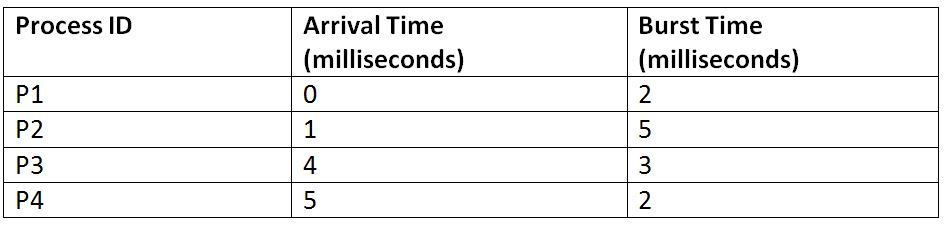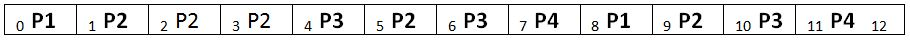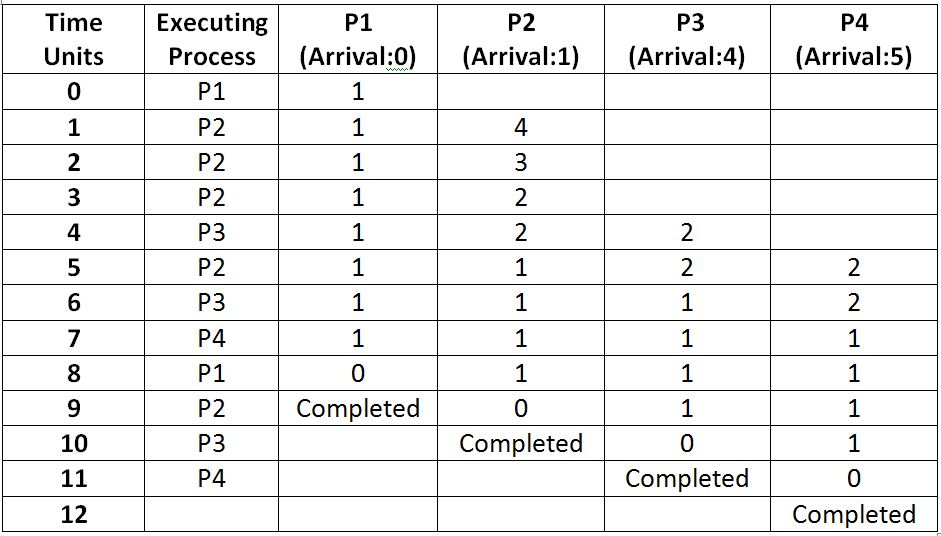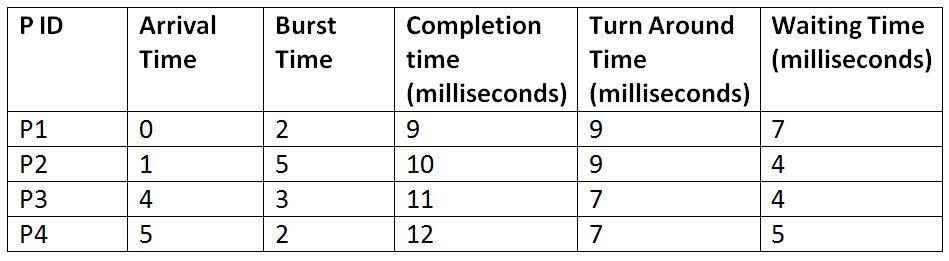# LRTF: Longest Remaining Time First Scheduling Algorithm

This article is about the Longest Remaining Time First (LRTF) scheduling algorithm used by the Operating system to schedule processes. In this article, we will study how this algorithm works. We will first describe its working and they will also look at an example for understanding it better.
Submitted by Monika Sharma, on June 23, 2019

LRTF, which stands for Longest Remaining Time First is a scheduling Algorithm used by the operating system to schedule the incoming processes so that they can be executed in a systematic way. This algorithm schedules those processes first which have the longest processing time remaining for completion. This algorithm can also be called as the preemptive version of the LJF scheduling algorithm. However, like the LJF, this algorithm is also not.

Let us take an example to understand it further. Suppose there are three processes with process Id's P1, P2, P3, and P4, and they have the following details:Gant Chart:Explanation: Each cell under the columns P1, P2, P3, and P4 denotes the remaining time required by that process for being completed.Note: Here, after all the process are left at the same time, then the execution order depends upon the arrival time. The process which has arrived first will be executed first in such cases.

Calculations:```    Total Turn Around Time = 9 + 9 + 7 + 7
= 32 milliseconds
Average Turn Around Time= Total Turn Around Time / Total No. of Processes
= 32 / 4
= 8 milliseconds

Total Waiting Time = 7 + 4 + 4 + 5
= 20 milliseconds
Average Waiting Time = Total Waiting Time / Total No. of Processes
= 20 / 4
= 5 milliseconds
```

Note: It can be observed that in spite of having much less burst time of each process, the average waiting time and average turn-around time is much high.